# Introduction to decimals#### Everything You Need in One Place

Homework problems? Exam preparation? Trying to grasp a concept or just brushing up the basics? Our extensive help & practice library have got you covered.#### Learn and Practice With Ease

Our proven video lessons ease you through problems quickly, and you get tonnes of friendly practice on questions that trip students up on tests and finals.#### Instant and Unlimited Help

Our personalized learning platform enables you to instantly find the exact walkthrough to your specific type of question. Activate unlimited help now!

0/3
##### Intros
###### Lessons
1. What are decimals?
2. How to use base ten (block) models to represent decimals
3. Standard, expanded, and written forms for decimals
0/15
##### Examples
###### Lessons
1. Naming decimal place values
Write the place value of the underlined digit in the decimal number.
1. 14.9
2. 357.2
3. 0.68
4. 4.21
2. Decimal digit values
Fill out the value of each digit in the boxes. Then, write the expanded and written forms for the decimal.
1.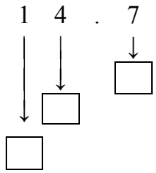2.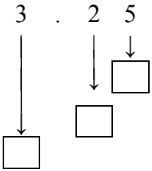3.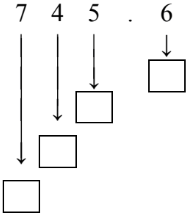4.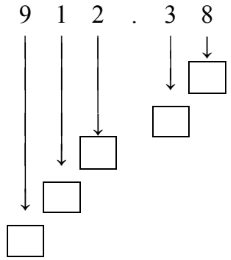3. Decimals represented by base ten (block) models
What is the decimal represented by the base ten (block) model?
1.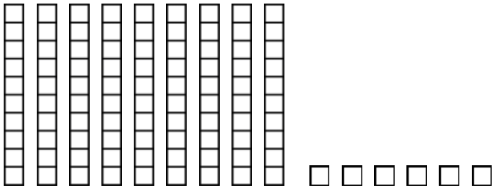2.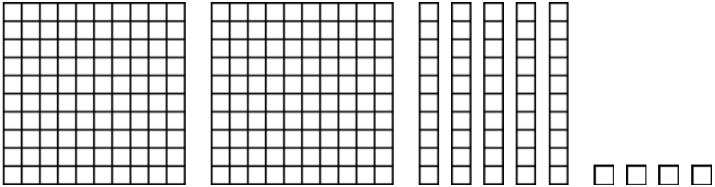3.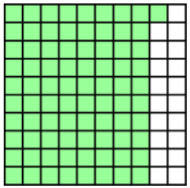4.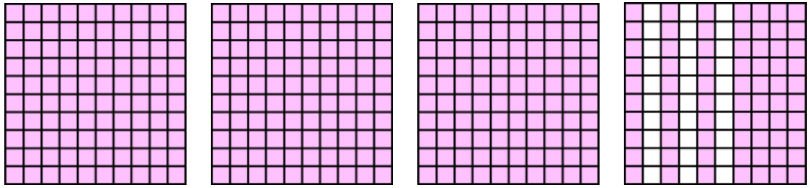4. Equivalent decimal tenths and hundredths
Using the list of decimals shown below:

0.60 $\enspace$ 0.04$\enspace$ 0.20$\enspace$ 0.5$\enspace$ 0.02$\enspace$ 0.40$\enspace$ 0.2$\enspace$ 0.8$\enspace$ 0.4$\enspace$ 0.31$\enspace$ 0.97$\enspace$ 0.06$\enspace$
1. Circle all equivalent pairs of decimals and write out number sentences using the equal (=) sign
2. Colour in a hundredths model block to represent each equivalent pair.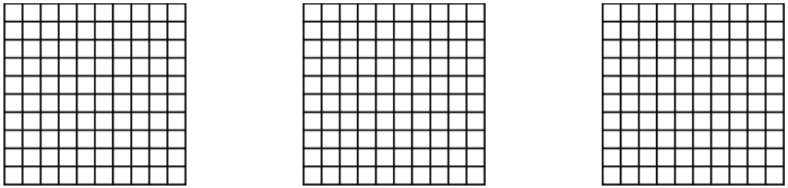3. Describe each pair of equivalent decimals from parts a and b using words (written as number of tenths vs. number of hundredths)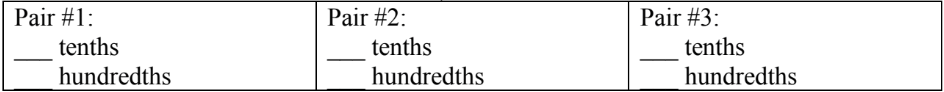0%
##### Practice
###### Topic Notes

In this lesson, we will learn:

• Decimals are numbers with place values even smaller than the ones place
• How to use base ten (block) models to represent a decimal's place values (ones place, tenths place, hundredths place) using hundred blocks, tens sticks, and single blocks
• How to write decimals in standard, expanded, and written forms.

Notes:

• Decimals are numbers with place values even smaller than the ones place.
• You can write a decimal point (.) at the end of any whole number
• Writing digits to the right of the decimal point gives decimal place values

• Ex. 1.5 shows decimal place of tenths; 2.64 shows decimal place of hundredths
• The names of decimal place values mirror the names of whole number place values, but you need to add the "-ths" suffix to the end.
• Each place value is 10 times MORE than the place on its right
• Each place value is 10 times LESS than the place on its left

• Ex. 111.11

 hundreds tens one . tenths hundredths 1 1 1 . 1 1 =100 =10 =1 =0.1 =0.01

• Decimals can be represented with base ten (block) models
• "One whole" or hundred block = ones place value
• Column or stick = tenths place value
• Single square = hundredths place value

• Ex. 1.25• Decimals can be written in standard, expanded, and written forms:
• Standard form: the decimal is written with number digits and a decimal point
• Expanded form: the decimal is written as a sum of values for all place values
• Written form: the decimal is written with words naming digits and place values from left to right

• Ex. 12.34 (read aloud as "twelve point three four")

 Standard form: 12.34 Expanded form: 10 + 2 + 0.3 + 0.04 Written form: twelve and three tenths four hundredths OR twelve and thirty-four hundredths

• Trailing zeroes (at the end/right of the number) are NOT important in decimals
• Ex. 0.5 and 0.50 are equal (5 tenths = 50 hundredths)